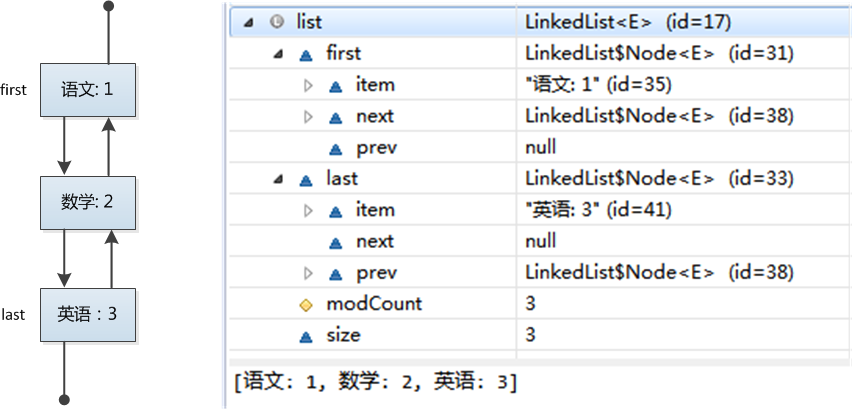## 一、 概述

Doubly-linked list implementation of the List and Deque interfaces. Implements all optional list operations, and permits all elements (including null).

``````LinkedList<String> list = new LinkedList<String>();
``````### 二、 set和get函数

``````public E set(int index, E element) {
checkElementIndex(index);
Node<E> x = node(index);
E oldVal = x.item;
x.item = element;
return oldVal;
}
public E get(int index) {
checkElementIndex(index);
return node(index).item;
}
``````

``````Node<E> node(int index) {
// assert isElementIndex(index);
if (index < (size >> 1)) {
Node<E> x = first;
for (int i = 0; i < index; i++)
x = x.next;
return x;
} else {
Node<E> x = last;
for (int i = size - 1; i > index; i--)
x = x.prev;
return x;
}
}
``````